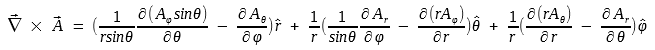# Curl

The curl of a vector field is a measure of the field's tendency to circulate about a point. James Clerk Maxwell invented the term "curl".

Curl is a point function

### Circulation

Circulation (the figure below) is defined as a line integral of the vector field A over a closed path C: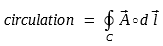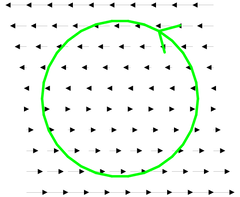### Curl

Curl at a specific point is defined as the circulation per unit area over an infinitesimal path around that point.where C is a path around the point of interest and the ΔS is the surface area enclosed by that path.

Cur is a vector quantity and its direction is the normal direction (perpendicular) of the surface ΔS where the circulation is a maximum.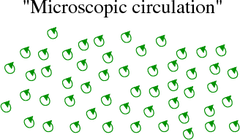### Curl Example

The field lines in the figure below represent the flow lines of water in a river.

Let's look for points at which the flow vectors on one side of the point are significantly different (in magnitude, direction, or both) from the flow vectors on the opposite side of the point.Imagine putting a tiny disc at each point in the flow. If the flow would cause the disc to rotate, the center of the disc marks a point of nonzero curl.

The direction of the curl is along the axis of the disc which is determined by the right-hand rule: if you curl the fingers of your right hand along the circulation, your thumb points in the direction of a positive curl.

Thus we can find that the points 1, 2, and 3 are high-curl locations.

### Curl Calculation Formula

Curl can be obtained by using the "del cross"operator.

Curl in Cartesian coordinates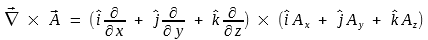The vector cross-product may be written as a determinant: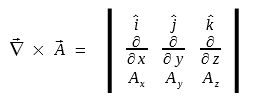This can be expanded to: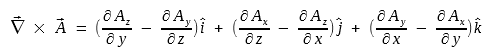Each component (in the parentheses) of the curl of vector field A indicates the tendency of the field to rotate in one of the coordinate planes.
If the curl of the field at a point has a large x-component, it means that the field has significant circulation about that point in the y-z plane.

Curl in cylindrical coordinatesCurl in spherical coordinates